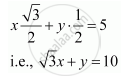CBSE (Arts) Class 11CBSE
Share

# Find the Equation of the Line Which is at a Perpendicular Distance of 5 Units from the Origin and the Angle Made by the Perpendicular with the Positive X-axis is 30° - CBSE (Arts) Class 11 - Mathematics

ConceptVarious Forms of the Equation of a Line

#### Question

Find the equation of the line which is at a perpendicular distance of 5 units from the origin and the angle made by the perpendicular with the positive x-axis is 30°

#### Solution

If is the length of the normal from the origin to a line and ω is the angle made by the normal with the positive direction of the x-axis, then the equation of the line is given by xcos ω + sin ω = p.

Here, p = 5 units and ω = 30°

Thus, the required equation of the given line is

x cos 30° + y sin 30° = 5Is there an error in this question or solution?

#### Video TutorialsVIEW ALL 

Solution Find the Equation of the Line Which is at a Perpendicular Distance of 5 Units from the Origin and the Angle Made by the Perpendicular with the Positive X-axis is 30° Concept: Various Forms of the Equation of a Line.
S